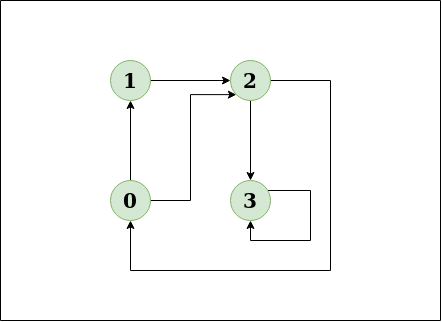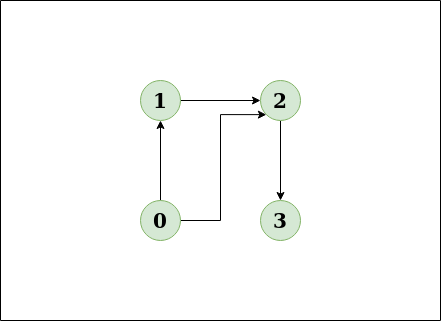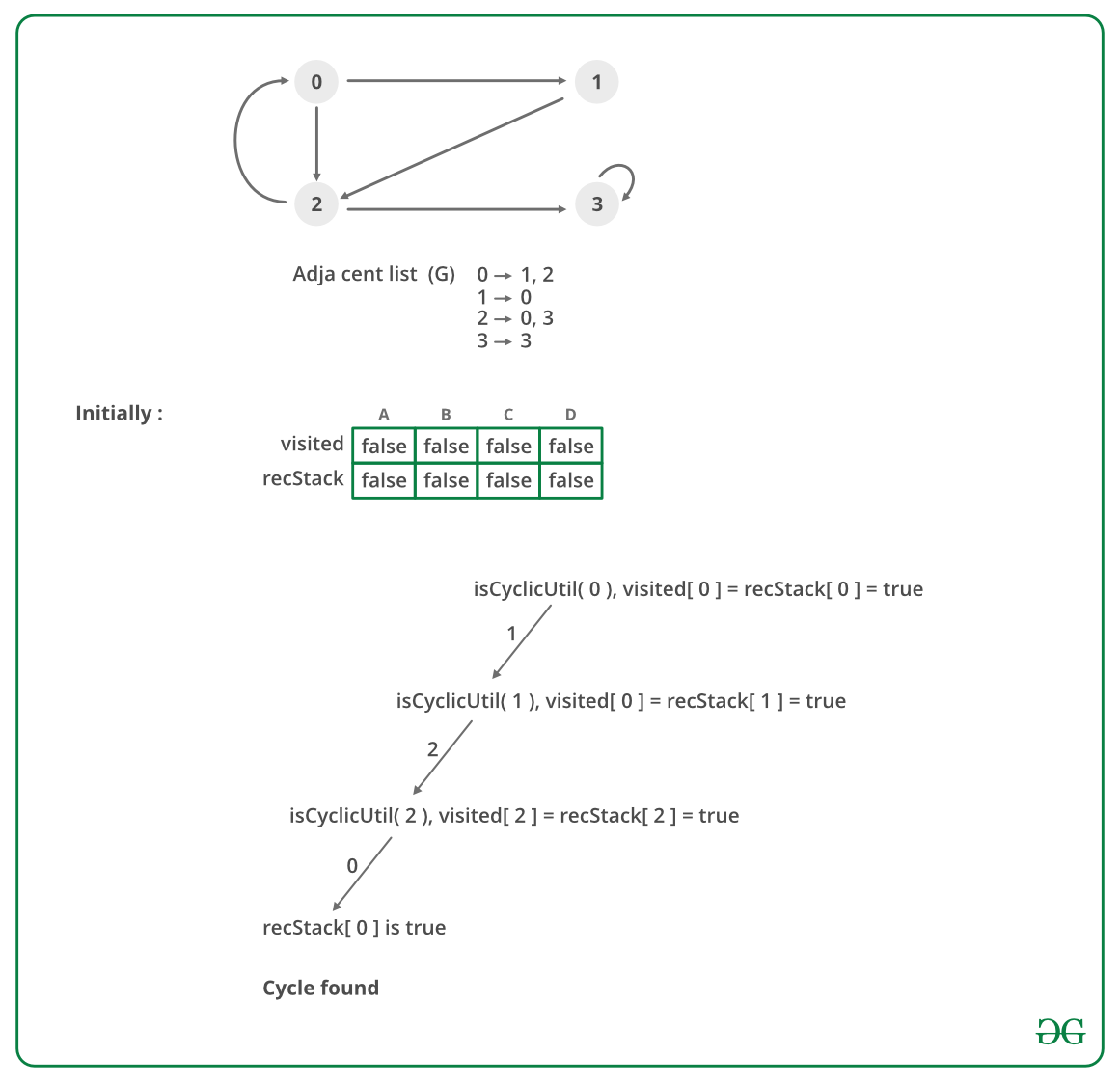Open in App
Not now

# Detect Cycle in a Directed Graph

• Difficulty Level : Medium
• Last Updated : 20 Dec, 2022

Given the root of a Directed graph, The task is to check whether the graph contains a cycle if yes then return true, return false otherwise.

Examples:

Input: N = 4, E = 6Output: Yes
Explanation: The diagram clearly shows a cycle 0 -> 2 -> 0

Input: N = 4, E = 4Output: No
Explanation: The diagram clearly shows no cycle

There is a cycle in a graph only if there is a back edge present in the graph. Depth First Traversal can be used to detect a cycle in a Graph, DFS for a connected graph produces a tree.

If the graph is disconnected then get the DFS forest and check for a cycle in individual trees by checking back edges. To detect a back edge, keep track of vertices currently in the recursion stack of function for DFS traversal. If a vertex is reached that is already in the recursion stack then there is a cycle in the tree.

Note: A Back edge is an edge that is from a node to itself (self-loop) or one of its ancestors in the tree produced by DFS. Thus the edge that connects the current vertex to the vertex in the recursion stack is a back edge.

Illustration:

In the following graph, there are 3 back edges, marked with a cross sign. Observe that these 3 back edges indicate 3 cycles present in the graph.Use recStack[] array to keep track of vertices in the recursion stack.

Dry run of the above approach:Follow the below steps to Implement the idea:

• Create the graph using the given number of edges and vertices.
• Create a recursive function that initializes the current vertex, visited array, and recursion stack.
• Mark the current node as visited and also mark the index in the recursion stack.
• Find all the vertices which are not visited and are adjacent to the current node and recursively call the function for those vertices
• If the recursive function returns true, return true.
• If the adjacent vertices are already marked in the recursion stack then return true.
• Create a wrapper function, that calls the recursive function for all the vertices, and
• If any function returns true return true
• Else if for all vertices the function returns false return false.

Below is the implementation of the above approach:

## C++

 `// A C++ Program to detect cycle in a graph` `#include`   `using` `namespace` `std;`   `class` `Graph` `{` `    ``int` `V;    ``// No. of vertices` `    ``list<``int``> *adj;    ``// Pointer to an array containing adjacency lists` `    ``bool` `isCyclicUtil(``int` `v, ``bool` `visited[], ``bool` `*rs);  ``// used by isCyclic()` `public``:` `    ``Graph(``int` `V);   ``// Constructor` `    ``void` `addEdge(``int` `v, ``int` `w);   ``// to add an edge to graph` `    ``bool` `isCyclic();    ``// returns true if there is a cycle in this graph` `};`   `Graph::Graph(``int` `V)` `{` `    ``this``->V = V;` `    ``adj = ``new` `list<``int``>[V];` `}`   `void` `Graph::addEdge(``int` `v, ``int` `w)` `{` `    ``adj[v].push_back(w); ``// Add w to v’s list.` `}`   `// This function is a variation of DFSUtil() in ` `// https://www.geeksforgeeks.org/archives/18212` `bool` `Graph::isCyclicUtil(``int` `v, ``bool` `visited[], ``bool` `*recStack)` `{` `    ``if``(visited[v] == ``false``)` `    ``{` `        ``// Mark the current node as visited and part of recursion stack` `        ``visited[v] = ``true``;` `        ``recStack[v] = ``true``;`   `        ``// Recur for all the vertices adjacent to this vertex` `        ``list<``int``>::iterator i;` `        ``for``(i = adj[v].begin(); i != adj[v].end(); ++i)` `        ``{` `            ``if` `( !visited[*i] && isCyclicUtil(*i, visited, recStack) )` `                ``return` `true``;` `            ``else` `if` `(recStack[*i])` `                ``return` `true``;` `        ``}`   `    ``}` `    ``recStack[v] = ``false``;  ``// remove the vertex from recursion stack` `    ``return` `false``;` `}`   `// Returns true if the graph contains a cycle, else false.` `// This function is a variation of DFS() in ` `// https://www.geeksforgeeks.org/archives/18212` `bool` `Graph::isCyclic()` `{` `    ``// Mark all the vertices as not visited and not part of recursion` `    ``// stack` `    ``bool` `*visited = ``new` `bool``[V];` `    ``bool` `*recStack = ``new` `bool``[V];` `    ``for``(``int` `i = 0; i < V; i++)` `    ``{` `        ``visited[i] = ``false``;` `        ``recStack[i] = ``false``;` `    ``}`   `    ``// Call the recursive helper function to detect cycle in different` `    ``// DFS trees` `    ``for``(``int` `i = 0; i < V; i++)` `        ``if` `( !visited[i] && isCyclicUtil(i, visited, recStack))` `            ``return` `true``;`   `    ``return` `false``;` `}`   `int` `main()` `{` `    ``// Create a graph given in the above diagram` `    ``Graph g(4);` `    ``g.addEdge(0, 1);` `    ``g.addEdge(0, 2);` `    ``g.addEdge(1, 2);` `    ``g.addEdge(2, 0);` `    ``g.addEdge(2, 3);` `    ``g.addEdge(3, 3);`   `    ``if``(g.isCyclic())` `        ``cout << ``"Graph contains cycle"``;` `    ``else` `        ``cout << ``"Graph doesn't contain cycle"``;` `    ``return` `0;` `}`

## Java

 `// A Java Program to detect cycle in a graph` `import` `java.util.ArrayList;` `import` `java.util.LinkedList;` `import` `java.util.List;`   `class` `Graph {` `    `  `    ``private` `final` `int` `V;` `    ``private` `final` `List> adj;`   `    ``public` `Graph(``int` `V) ` `    ``{` `        ``this``.V = V;` `        ``adj = ``new` `ArrayList<>(V);` `        `  `        ``for` `(``int` `i = ``0``; i < V; i++)` `            ``adj.add(``new` `LinkedList<>());` `    ``}` `    `  `    ``// This function is a variation of DFSUtil() in ` `    ``// https://www.geeksforgeeks.org/archives/18212` `    ``private` `boolean` `isCyclicUtil(``int` `i, ``boolean``[] visited,` `                                      ``boolean``[] recStack) ` `    ``{` `        `  `        ``// Mark the current node as visited and` `        ``// part of recursion stack` `        ``if` `(recStack[i])` `            ``return` `true``;`   `        ``if` `(visited[i])` `            ``return` `false``;` `            `  `        ``visited[i] = ``true``;`   `        ``recStack[i] = ``true``;` `        ``List children = adj.get(i);` `        `  `        ``for` `(Integer c: children)` `            ``if` `(isCyclicUtil(c, visited, recStack))` `                ``return` `true``;` `                `  `        ``recStack[i] = ``false``;`   `        ``return` `false``;` `    ``}`   `    ``private` `void` `addEdge(``int` `source, ``int` `dest) {` `        ``adj.get(source).add(dest);` `    ``}`   `    ``// Returns true if the graph contains a ` `    ``// cycle, else false.` `    ``// This function is a variation of DFS() in ` `    ``// https://www.geeksforgeeks.org/archives/18212` `    ``private` `boolean` `isCyclic() ` `    ``{` `        `  `        ``// Mark all the vertices as not visited and` `        ``// not part of recursion stack` `        ``boolean``[] visited = ``new` `boolean``[V];` `        ``boolean``[] recStack = ``new` `boolean``[V];` `        `  `        `  `        ``// Call the recursive helper function to` `        ``// detect cycle in different DFS trees` `        ``for` `(``int` `i = ``0``; i < V; i++)` `            ``if` `(isCyclicUtil(i, visited, recStack))` `                ``return` `true``;`   `        ``return` `false``;` `    ``}`   `    ``// Driver code` `    ``public` `static` `void` `main(String[] args)` `    ``{` `        ``Graph graph = ``new` `Graph(``4``);` `        ``graph.addEdge(``0``, ``1``);` `        ``graph.addEdge(``0``, ``2``);` `        ``graph.addEdge(``1``, ``2``);` `        ``graph.addEdge(``2``, ``0``);` `        ``graph.addEdge(``2``, ``3``);` `        ``graph.addEdge(``3``, ``3``);` `        `  `        ``if``(graph.isCyclic())` `            ``System.out.println(``"Graph contains cycle"``);` `        ``else` `            ``System.out.println(``"Graph doesn't "` `                                    ``+ ``"contain cycle"``);` `    ``}` `}`   `// This code is contributed by Sagar Shah.`

## Python

 `# Python program to detect cycle ` `# in a graph`   `from` `collections ``import` `defaultdict`   `class` `Graph():` `    ``def` `__init__(``self``,vertices):` `        ``self``.graph ``=` `defaultdict(``list``)` `        ``self``.V ``=` `vertices`   `    ``def` `addEdge(``self``,u,v):` `        ``self``.graph[u].append(v)`   `    ``def` `isCyclicUtil(``self``, v, visited, recStack):`   `        ``# Mark current node as visited and ` `        ``# adds to recursion stack` `        ``visited[v] ``=` `True` `        ``recStack[v] ``=` `True`   `        ``# Recur for all neighbours` `        ``# if any neighbour is visited and in ` `        ``# recStack then graph is cyclic` `        ``for` `neighbour ``in` `self``.graph[v]:` `            ``if` `visited[neighbour] ``=``=` `False``:` `                ``if` `self``.isCyclicUtil(neighbour, visited, recStack) ``=``=` `True``:` `                    ``return` `True` `            ``elif` `recStack[neighbour] ``=``=` `True``:` `                ``return` `True`   `        ``# The node needs to be popped from ` `        ``# recursion stack before function ends` `        ``recStack[v] ``=` `False` `        ``return` `False`   `    ``# Returns true if graph is cyclic else false` `    ``def` `isCyclic(``self``):` `        ``visited ``=` `[``False``] ``*` `(``self``.V ``+` `1``)` `        ``recStack ``=` `[``False``] ``*` `(``self``.V ``+` `1``)` `        ``for` `node ``in` `range``(``self``.V):` `            ``if` `visited[node] ``=``=` `False``:` `                ``if` `self``.isCyclicUtil(node,visited,recStack) ``=``=` `True``:` `                    ``return` `True` `        ``return` `False`   `g ``=` `Graph(``4``)` `g.addEdge(``0``, ``1``)` `g.addEdge(``0``, ``2``)` `g.addEdge(``1``, ``2``)` `g.addEdge(``2``, ``0``)` `g.addEdge(``2``, ``3``)` `g.addEdge(``3``, ``3``)` `if` `g.isCyclic() ``=``=` `1``:` `    ``print` `"Graph contains cycle"` `else``:` `    ``print` `"Graph doesn't contain cycle"`   `# Thanks to Divyanshu Mehta for contributing this code`

## C#

 `// A C# Program to detect cycle in a graph ` `using` `System;` `using` `System.Collections.Generic;`   `public` `class` `Graph { ` `    `  `    ``private` `readonly` `int` `V; ` `    ``private` `readonly` `List> adj; `   `    ``public` `Graph(``int` `V) ` `    ``{ ` `        ``this``.V = V; ` `        ``adj = ``new` `List>(V); ` `        `  `        ``for` `(``int` `i = 0; i < V; i++) ` `            ``adj.Add(``new` `List<``int``>()); ` `    ``} ` `    `  `    ``// This function is a variation of DFSUtil() in ` `    ``// https://www.geeksforgeeks.org/archives/18212 ` `    ``private` `bool` `isCyclicUtil(``int` `i, ``bool``[] visited, ` `                                    ``bool``[] recStack) ` `    ``{ ` `        `  `        ``// Mark the current node as visited and ` `        ``// part of recursion stack ` `        ``if` `(recStack[i]) ` `            ``return` `true``; `   `        ``if` `(visited[i]) ` `            ``return` `false``; ` `            `  `        ``visited[i] = ``true``; `   `        ``recStack[i] = ``true``; ` `        ``List<``int``> children = adj[i]; ` `        `  `        ``foreach` `(``int` `c ``in` `children) ` `            ``if` `(isCyclicUtil(c, visited, recStack)) ` `                ``return` `true``; ` `                `  `        ``recStack[i] = ``false``; `   `        ``return` `false``; ` `    ``} `   `    ``private` `void` `addEdge(``int` `sou, ``int` `dest) { ` `        ``adj[sou].Add(dest); ` `    ``} `   `    ``// Returns true if the graph contains a ` `    ``// cycle, else false. ` `    ``// This function is a variation of DFS() in ` `    ``// https://www.geeksforgeeks.org/archives/18212 ` `    ``private` `bool` `isCyclic() ` `    ``{ ` `        `  `        ``// Mark all the vertices as not visited and ` `        ``// not part of recursion stack ` `        ``bool``[] visited = ``new` `bool``[V]; ` `        ``bool``[] recStack = ``new` `bool``[V]; ` `        `  `        `  `        ``// Call the recursive helper function to ` `        ``// detect cycle in different DFS trees ` `        ``for` `(``int` `i = 0; i < V; i++) ` `            ``if` `(isCyclicUtil(i, visited, recStack)) ` `                ``return` `true``; `   `        ``return` `false``; ` `    ``} `   `    ``// Driver code ` `    ``public` `static` `void` `Main(String[] args) ` `    ``{ ` `        ``Graph graph = ``new` `Graph(4); ` `        ``graph.addEdge(0, 1); ` `        ``graph.addEdge(0, 2); ` `        ``graph.addEdge(1, 2); ` `        ``graph.addEdge(2, 0); ` `        ``graph.addEdge(2, 3); ` `        ``graph.addEdge(3, 3); ` `        `  `        ``if``(graph.isCyclic()) ` `            ``Console.WriteLine(``"Graph contains cycle"``); ` `        ``else` `            ``Console.WriteLine(``"Graph doesn't "` `                                    ``+ ``"contain cycle"``); ` `    ``} ` `} `   `// This code contributed by Rajput-Ji`

## Javascript

 `// A JavaScript Program to detect cycle in a graph`   `let V;` `let adj=[];` `function` `Graph(v)` `{` `    ``V=v;` `    ``for` `(let i = 0; i < V; i++)` `        ``adj.push([]);` `}`   `// This function is a variation of DFSUtil() in` `    ``// https://www.geeksforgeeks.org/archives/18212` `function` `isCyclicUtil(i,visited,recStack)` `{` `    ``// Mark the current node as visited and` `        ``// part of recursion stack` `        ``if` `(recStack[i])` `            ``return` `true``;` ` `  `        ``if` `(visited[i])` `            ``return` `false``;` `             `  `        ``visited[i] = ``true``;` ` `  `        ``recStack[i] = ``true``;` `        ``let children = adj[i];` `         `  `        ``for` `(let c=0;c< children.length;c++)` `            ``if` `(isCyclicUtil(children, visited, recStack))` `                ``return` `true``;` `                 `  `        ``recStack[i] = ``false``;` ` `  `        ``return` `false``;` `}`   `function` `addEdge(source,dest)` `{` `    ``adj .push(dest);` `}`   `// Returns true if the graph contains a` `    ``// cycle, else false.` `    ``// This function is a variation of DFS() in` `    ``// https://www.geeksforgeeks.org/archives/18212` `function` `isCyclic()` `{` `    ``// Mark all the vertices as not visited and` `        ``// not part of recursion stack` `        ``let visited = ``new` `Array(V);` `        ``let recStack = ``new` `Array(V);` `        ``for``(let i=0;i

Output

`Graph contains cycle`

Time Complexity: O(V+E), the Time Complexity of this method is the same as the time complexity of DFS traversal which is O(V+E).
Auxiliary Space: O(V). To store the visited and recursion stack O(V) space is needed.

In the below article, another O(V + E) method is discussed :
Detect Cycle in a direct graph using colors

My Personal Notes arrow_drop_up
Related Articles